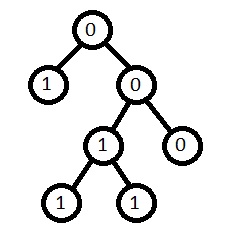# Count Univalue Subtrees

Posted: 8 Sep, 2020
Difficulty: Moderate

## PROBLEM STATEMENT

#### For example: for the binary tree, given in the following diagram, the number of unival trees is 5.##### Input Format:
``````The first line of input contains an integer T, the number of test cases.

The next T lines, where each line contains elements in the level order form. The input consists of values of nodes separated by a single space in a single line. In case a node is null, we take -1 on its place.

For example, the input for the tree depicted in the below image would be :
````````````1
2 3
4 -1 5 6
-1 7 -1 -1 -1 -1
-1 -1
``````

#### Explanation :

``````Level 1 :
The root node of the tree is 1

Level 2 :
Left child of 1 = 2
Right child of 1 = 3

Level 3 :
Left child of 2 = 4
Right child of 2 = null (-1)
Left child of 3 = 5
Right child of 3 = 6

Level 4 :
Left child of 4 = null (-1)
Right child of 4 = 7
Left child of 5 = null (-1)
Right child of 5 = null (-1)
Left child of 6 = null (-1)
Right child of 6 = null (-1)

Level 5 :
Left child of 7 = null (-1)
Right child of 7 = null (-1)

The first not-null node (of the previous level) is treated as the parent of the first two nodes of the current level. The second not-null node (of the previous level) is treated as the parent node for the next two nodes of the current level and so on.
The input ends when all nodes at the last level are null (-1).
``````
##### Note :
``````The above format was just to provide clarity on how the input is formed for a given tree.
The sequence will be put together in a single line separated by a single space. Hence, for the above depicted tree, the input will be given as:

1
1 2 3 4 -1 5 6 -1 7 -1 -1 -1 -1 -1 -1
``````
##### Output Format:
``````For every test case print single line containing an integer i.e the count of unival trees.
``````

#### Note

``````You do not need to print anything, it has already been taken care of. Just implement the given function.
``````
##### Constraints:
``````1 <= T <= 10
0 <= N <= 10^5
0 <= data <= 10^4, where data is the value for a node.

Time Limit: 1sec
``````Approach 1

A brute force approach is to traverse all nodes of the tree. For each node, we will calculate the number of unival subtrees recursively from the left and right subtrees of the current node, as count. Now we will check all nodes in the subtree of the current node using the either recursive or iterative method. If all the subnodes have value equals the current node value then we will increment the count.